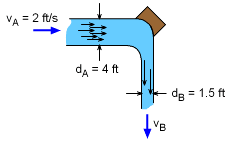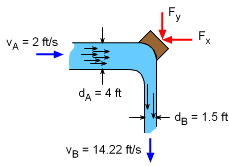Ch 4. Particle Momentum and Impulse Multimedia Engineering Dynamics Impulse & Momentum Consv. Linear Momentum Impact AngularMomentum MassFlow
 Chapter - Particle - 1. General Motion 2. Force & Accel. 3. Energy 4. Momentum - Rigid Body - 5. General Motion 6. Force & Accel. 7. Energy 8. Momentum 9. 3-D Motion 10. Vibrations Appendix Basic Math Units Basic Equations Sections Search eBooks Dynamics Fluids Math Mechanics Statics Thermodynamics Author(s): Kurt Gramoll ©Kurt GramollDYNAMICS - EXAMPLEWater Flow Direction Change Example A 4 ft diameter pipe is supported by a block as it makes a 90o turn. What is the force in the y-direction on the block due to the water direction change? Assume the specific weight, γw, of the water is 62.4 lb/ft3. SolutionForces Due to Flow Momentum Change To determine the change in momentum, fluid velocity needs to be determined for the out flow. Using the conservation of mass equation,      vAAA = vBAB      (2) [(4/2)2π] = vB [(1.5/2)2π]      8 = vB 0.752      vB = 14.22 ft/s The mass flow rate can be calculated using the 4 ft or the 1.5 ft pipe,     dm/dt =ρvAAA (= ρvBAB)        = (γ/g) [(4/2)2π]        = (62.4/32.2) 12.57        = 48.70 slug/s The vertical reaction force must equal the change of momentum in the vertical direction,       Fy = dm/dt (vBy - vAy)            = 48.70 (14.22 - 0) Fy = 692.7 lb

Practice Homework and Test problems now available in the 'Eng Dynamics' mobile app
Includes over 400 problems with complete detailed solutions.
Available now at the Google Play Store and Apple App Store.